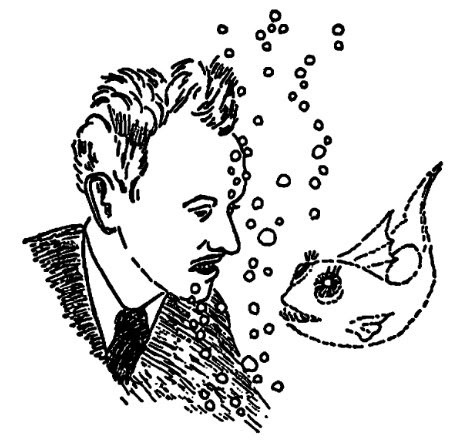### Paul Dirac and the relativistic world

posted by @ulaulaman #PaulDirac #DiracDay #DiracEquation #Klein-GordonEquation #relativity #physics
It seems to be one of the fundamental features of nature that fundamental physical laws are described in terms of a mathematical theory of great beauty and power, needing quite a high standard of mathematics for one to understand it.
(Paul Dirac)For a physicists the beauty of an equation is often in its conciseness and in the amount of physical information that is able to synthesize, and so the wide variety of systems that it can be represented. For example, the Schrodinger equation, in its most synthetic form, is able to describe a disparate number of systems, and only descending the details of each system, physicists are able to describe the system with with more or less complicated solutions. $i \frac{\partial}{\partial t} \psi = H \psi$ Something like that it's maked by the equations discovered by Klein-Gordon and Dirac, but for the relativistic motion: indeed particles are able to travel even at near the speed of light, and in that case the Schrodinger equation is no longer sufficient to describe their dynamics.
From this we need to describe the world from the point of view of quantum relativistic: the Klein-Gordon equation is born: $\partial^\mu \partial_\mu \phi + m^2 \phi = 0$ It has two drawbacks: negative energy as a solution and interpretation of the wave function. If, as in quantum mechanics, we associate the wave function with probability, or rather the probability's density, it happens that this can also take on negative values. At this point arrived the Dirac equation: $\left ( i \gamma^\mu \partial_\mu - m \right ) \psi = 0$ Thanks to the Dirac equation, whose one of the first success was the explanation of half-integer spin of the electron, we now have two fundamental tools: the first for the description of bosons (particles with integer spin), the second for the description of fermions (half-integer spin particles).
Read also: The Relation between Mathematics and Physics, a lecture by Dirac
In the image: Paul Dirac by George Gamow